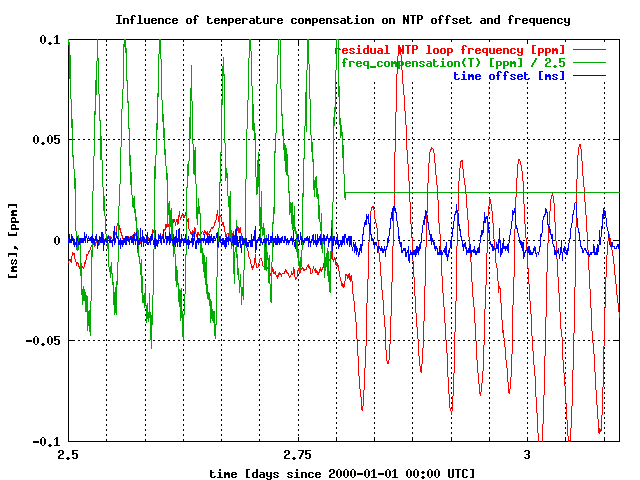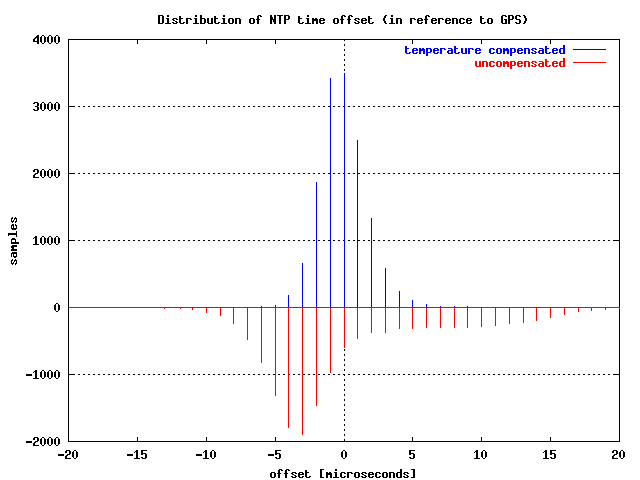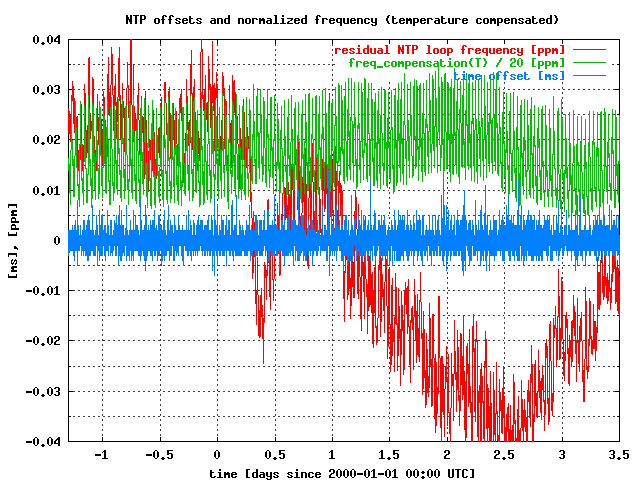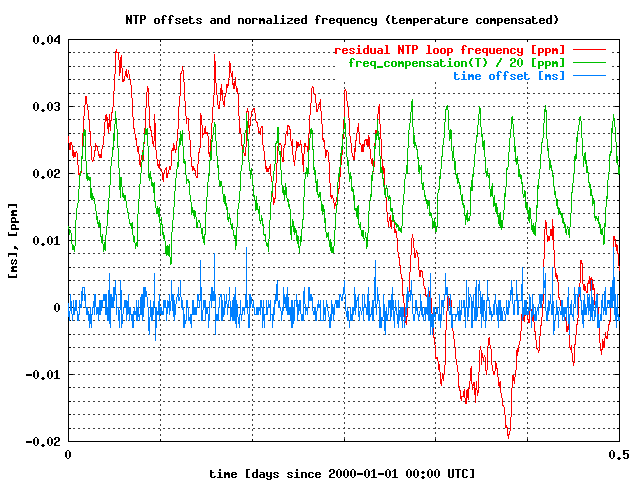# NTP temperature compensation

Mark Martinec, 2001-01-08

## Introduction

An experiment with NTP was set up having in mind the following facts:

• typical computer clock is implemented by an uncompensated quartz or SAW oscillator;
• the biggest influence on the frequency of such oscillator are changes in temperature;
• NTP phase locked loop has slow response in comparison to the rate of changes in temperature (at least in our environment);
• the time offset error is a function of the PLL response and the rate of change of the frequency, which may be quite fast in an air-conditioned environment (e.g. one temperature cycle of one degree Centigrade peak-to-peak every half an hour, in our case).

The purpose of the experiment was to determine to what degree the computer clock's frequency can be stabilized and time precision increased (deviation of time offsets decreased) by performing software-based temperature compensation of the oscillator's frequency.

## Model

First the temperature coefficient k1 of the computer clock's oscillator was roughly estimated by comparing room temperature changes to the changes of the normalized frequency offset (called drift by NTP). Later the estimate was improved in few iterations.

The stabilization mechanism used consists of a process (running a program in Perl) which is constantly measuring temperature near the oscillator, estimating frequency correction needed, and feeding this frequency correction to NTP's module ntp_loopfilter.c, where it it added to the NTP's drift_comp. The resulting sum is used by NTP to control the computer clock frequency. This effectively relieves NTP of the burden of compensating for the predictable fast-changing temperature-induced frequency error component, leaving only smaller residual frequency errors (random, or at least not easily predictable) for its mechanisms to compensate.

Although temperature characteristics of a quartz oscillator are nonlinear and may even show hysteresis, in our case large temperature range compensation is not needed. Frequency correction for a small range of temperatures (a few degrees Centigrade about average temperature) was first linearly approximated by a temperature coefficient k1:

freq_compensation = k0 + k1 . (T-28.1)

where:

k0 = 0 [ppm] ... (DC component is left for NTP to compensate)
k1 = 0.277 [ppm/K] ... is a linear temperature coefficient;
T ... is a temperature near the oscillator, in deg C;
freq_compensation [ppm] ... is the value to be summed with the NTP's drift_comp.

28.1 was chosen to produce average frequency correction of zero (k0 = 0) near the average oscillator temperature -- mostly for aesthetic reasons.

Later the model was slightly improved by adding a second-order component:

freq_compensation = k0 + k1 . (T-28.1) + k2 . (T-28.1)2;

where:

k0 and k1 are as above;
k2 = 0.012 [ppm/K2]

This served to slightly extend the temperature range where oscillator's frequency is adequately temperature-stabilized.

It is estimated that k1 determined for this particular host is accurate to few percent, and k2 is just a rough approximation.

## Results

The following diagram shows time offset and normalized frequency offset (called drift by NTP) as reported by NTP in the loopstats file. NTP's external clock reference was a GPS receiver, polling interval was fixed at 16 seconds, PPS signal was not used.

The first half of the diagram shows temperature-compensated NTP, the second half is the normal NTP behavior after turning off externally applied frequency corrections.

The green trace shows the applied normalized frequency offset correction, the freq_compensation, which is calculated as described above by an external process and added to the NTP's controlling frequency. The green trace is scaled-down by a factor of 2.5 to fit the diagram and to approximately match the amplitude of the red trace.

The direct cause of the increase of time offsets fluctuations after the compensation has been turned off is the relatively large PLL time constant. Instead of the optimal frequency compensation, which would be observed had the frequency changes been much slower, the large PLL time constant causes about 2.5-times smaller frequency correction in our particular case (30 minute temperature cycles).Figure 1.

## Distribution of time offsets

A distribution of time offsets is shown as a bihistogram. Temperature compensated NTP is shown above x-axis, uncompensated NTP is shown inverted below the x-axis. Each case represents about 14500 offset samples from the log file taken during two, slightly less than 5-day, intervals).Figure 2.

NOTE 1: discrete offset values (step = 1 us) are a result of the system clock resolution (microkernel).

NOTE 2: The skewness of the lower histogram (uncompensated oscillator) is due to the fact that during air-conditioning cycle in our computer room, the temperature rises faster during air-warming period, than the rate at which it is falling when air is being cooled.

## Some statistical measures for time offsets:

Uncompensated:

(14584 samples, 4.75 days)

median: -2.00 us
mean: 0.03 us
standard deviation: 6.30 us
10-percentile: -6.0 us
90-percentile: 10.0 us

Temperature compensated:

(14656 samples, 4.8 days)

median: 0.00 us
mean: -0.07 us
standard deviation: 1.78 us
10-percentile: -2.0 us
90-percentile: 2.0 us

## Conclusion

By using temperature compensation, the standard deviation of time offsets is reduced 3.54-times and the 10..90% percentile range of time offsets is reduced from 16 us to 4 us. I consider this a good yield for the addition of less then \$20 worth of hardware (1-Wire temperature sensor and a serial line adapter).

The described mechanism is now in regular use at both public NTP servers at the Institute "Josef Stefan".

## Appendix A - The Setup

 computer hardware: Compaq AlphaPC 164 432 operating system: Tru64 Unix 4.0B with MICRO_TIME kernel option ntpd version: modified ntpd 4.0.99k11 (should work with previous V4 versions as well) reference clock: Trimble Palisade GPS time receiver temperature probe accuracy: about 0.5 deg C temperature probe resolution: about 0.01 deg C temperature probe type: Dallas Semiconductors 1-Wire DS1820 temperature sensors with DS2480 serial line adapter

## Appendix B

A five day trace of temperature compensated NTP behavior:Figure 3.

NOTE: in this and in the following diagram the freq_compensation green trace (in [ppm]) is scaled down by a factor of 20.

A detail of the above:Figure 4.

On a related topic: http://www.ijs.si/time/